# Solution assignment 02 Root equations

### Assignment 2

Solve: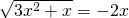### Solution

We can follow two paths. Firstly, we can determine for which values ofthe equation above is defined. In this case, both the expression under the root and the right-hand side have be to greater than or equal to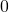. In this case this requires quite a few calculations.
We can also first try to figure out the solutions and then determine whether they satisfy the equation. Here we choose the second approach.

We square both sides of the equation and get: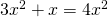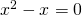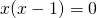This equation has the following solutions: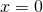or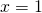We must now examine whether these solutions satisfy the original equation. We see thatdoes satisfy, butdoes not because a root can not have a negative value. Therefore, the solution of the original equation is: